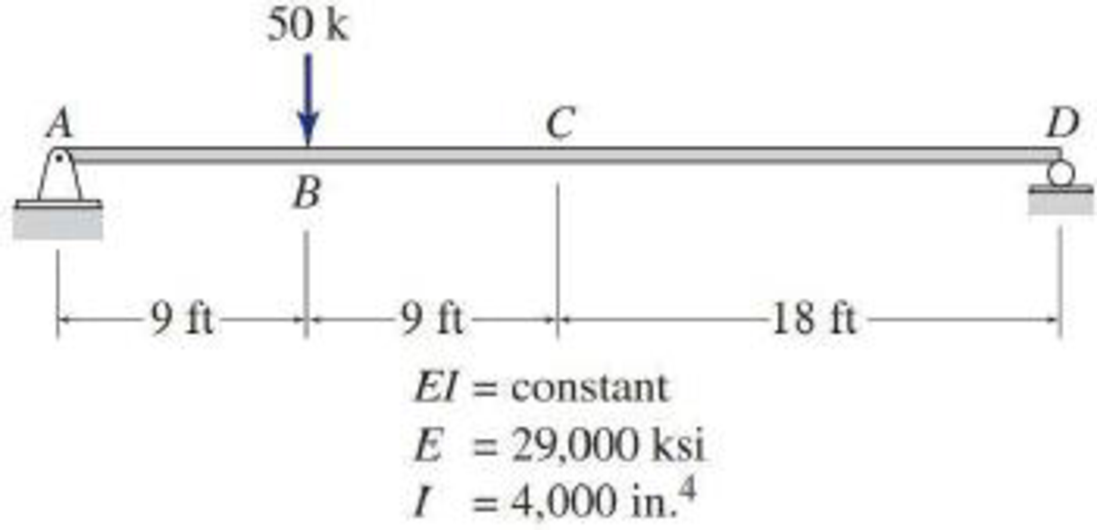# 6.40 through 6.43 Use the Conjugate-beam method to determine the slopes and deflections at points B and C of the beams Figs. P6.14 through P6.17. FIG. P6.17, P6.43

#### Solutions

Chapter
Section
Chapter 6, Problem 43P
Textbook Problem
268 views

## 6.40 through 6.43 Use the Conjugate-beam method to determine the slopes and deflections at points B and C of the beams Figs. P6.14 through P6.17.FIG. P6.17, P6.43To determine

Find the slope θB&θC and deflection ΔB&ΔC at point B and C of the given beam using the conjugate-beam method.

### Explanation of Solution

The Young’s modulus (E) is 29,000 ksi.

The moment of inertia (I) is 4,000in.4.

Calculation:

Consider flexural rigidity EI of the beam is constant.

Show the given beam as in Figure (1).

Refer Figure (1),

Consider upward is positive and downward is negative.

Consider clockwise is negative and counterclowise is positive.

Determine the support reaction at A using the Equation of equilibrium;

MD=0RA×36(50×27)=0RA=1,35036RA=37.5kips

Determine the reaction at support D;

V=0RA+RD=50RD=5037.5RD=12.5kips

Show the free body diagram of the given beam as in Figure (2).

Refer Figure (2),

Determine the bending moment at A;

MA=(12.5×36)(50×9)=450450=0

Determine the bending moment at B;

MB=37.5×9=337.5kipsft

Determine the bending moment at C;

MC=37.5×18(50×9)=225kipsft

Determine the moment at D;

MD=(37.5×36)(50×27)=1,3501,350=0

Show the M/EI diagram for the given beam as in Figure (3).

Show the M/EI diagram for the conjugate-beam as in Figure (4).

Consider counterclockwise is positive and clockwise is negative.

Determine the support reaction at A using the Equation of equilibrium;

RA(36)12(337.5EI)(9)(13×9+27)12(337.5EI)(27)(13×27+9)=0RA=127,575kips-ft236EIRA=3,543.75kips-ft2EI

Consider upward is positive and downward is negative.

V=0+RD3,543.75EI+12(337.5EI)(36)=0RD=2,531.75kips-ft2EI

Determine the shear force at B using the relation;

SB=RA+(12×b1×h1)

Here, b1 is the width and h1 is the height of the triangle,

Substitute 3,543.75kips-ft2EI for RA, 9 ft for b1, and 337.5EI for h1.

SB=3,543.75kips-ft2EI+(12×9×337.5EI)=2,025kips-ft2EI

Determine the deflection at point B using the relation;

θB=2,025kips-ft2EI

Substitute 29,000 ksi for E and 4,000in.4 for I.

Hence, the slope at point B is 0.0025rad_.

The bending moment at B in the conjugate beam is equal to defection at B on the real beam.

Determine the bending moment B using the relation;

MB=RA(b1)+[(12×b2×h2)(13×b2)]

Here, b1 is the distance between A and B, b2 is the width of triangle, and h2 is the height of the triangle

### Still sussing out bartleby?

Check out a sample textbook solution.

See a sample solution

#### The Solution to Your Study Problems

Bartleby provides explanations to thousands of textbook problems written by our experts, many with advanced degrees!

Get Started

Find more solutions based on key concepts
As we explained in earlier chapters, the air resistance to the motion of a vehicle is something important that ...

Engineering Fundamentals: An Introduction to Engineering (MindTap Course List)

What strategies e Wal-Mart and Loweâ€™s using to gain more online customers?

Systems Analysis and Design (Shelly Cashman Series) (MindTap Course List)

What does the kernel do?

Enhanced Discovering Computers 2017 (Shelly Cashman Series) (MindTap Course List)

How is cost of goods sold determined?

Cornerstones of Financial Accounting

Briefly describe a tombstone used for CNC workholding.

Precision Machining Technology (MindTap Course List)

If your motherboard supports ECC DDR3 memory, can you substitute non-ECC DDR3 memory?

A+ Guide to Hardware (Standalone Book) (MindTap Course List)

What is meant by a welder's duty cycle?

Welding: Principles and Applications (MindTap Course List)